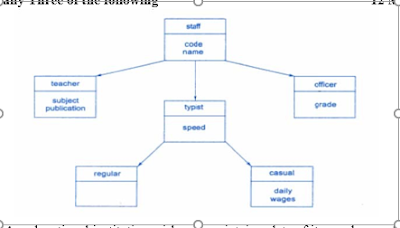## Q.1 Attempt any Five of the following                                                                  10 Marks

A.            Explain with suitable example, syntax of for loop in C++.
B.            Explain concept of abstract class.
C.            State the use of scope resolution operator and its use in C++.
D.            State use of new operator.
E.            List and explain use of any four file mode parameters       F.        Explain use of friend function with the help of suitable example.
G.     Write a C++ program to swap two numbers using pointer

## Q.2 Attempt any Three of the following                                                           12 Marks

A.  Write a C++ program that replaces the string “Computer” in the String “Diploma in Computer Engineering” with string “Information Technology”.

B.  Define classes to appropriately represent class hierarchy as shown in above figure.Use constructors for both classes and display Salary for a particular employee.

C.  Define a class named ‘Train’ representing following members:

## Data members :- -     Train Number

-         Train Name
-         Source
-         Destination
-         Journey Date
-         Capacity
Member functions:
-         Initialise members
-         Input Train data
-         Display data
Write a C++ program to test the train class.
D.  State any four points of differentiation between function overloading and function overriding.

## Q.3 Attempt any Three of the following                                                           12 Marks

A.          Write a C++ program to calculate sum of distance and display the results using friend function.
B.          Write a program to count no of occurrence of particular character  in text file
a.    Define concept of virtual base class.
b.    Give reason for assigning protected visibility specifier to a class member. D.  State rules of operator overloading.

## Q.4 Attempt any Three of the following                                                           12 Marks

A. An educational institution wishes to maintain a data of its employees. The hierarchical relationships of related classes are as shown in figure. Define all the classes to represent above hierarchy and define functions to retrieve individual information as and when required## Q.5 Attempt any Two of the following                                                             12 Marks

A.            1. State any four points of differentiation between compile time polymorphism and run time polymorphism
B.            i) Write a program that will create data file containing the list of telephone numbers as:
John 34567
Hari 56788 ……
Use a class object to store each set of data.
C.            Define a class named ‘Bank Account’ to represent following members:
Data members :-
-         Account Number
-         Name of Depositor
-         Account Type
-         Balance Amount
Member functions:
-         Initialize members
-         Deposit Amount
-         Withdraw Amount
-         Display Balance
Write a C++ program to test the Bank Account class for 10 customers.

## Q.6 Attempt any Two of the following                                                             12 Marks

A.          Give syntax of and explain various functions related to ifstream and ofstream classes: seekp(), getline(),hide(),tail()
B.          Fix the  compilation errors and find the output of following program  #include<iostream.h>
class Test { private: int x; public:
Test(int x = 0) { this->x = x; } void change(Test *t) { this = t; }
void print() { cout << "x = " << x << endl; }
}; void main() {
Test obj(5);
Test *ptr = new Test (10); obj.change(ptr); obj.print();
}
C.  A program uses a function named convert() in addition to its main function. The function main() declares the variable x within its body and the function convert declares two variables y and z within its body, z is made static. A fourth variable m is declared ahead(ie at top) of both the functions. State the visibility and lifetime of each of these variables.

1.2.3.4.